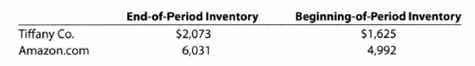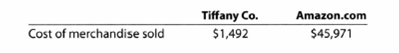Chapter 7, Problem 7.5CP### Accounting (Text Only)

26th Edition
Carl Warren + 2 others
ISBN: 9781285743615

#### Solutions

Chapter
Section### Accounting (Text Only)

26th Edition
Carl Warren + 2 others
ISBN: 9781285743615
Textbook Problem
10 views

# Comparing inventory ratios for two companiesTiffany Co. is a high-end jewelry retailer, while Amazon.com uses its e-commerce services, features, and technologies to sell its products through the Internet. Recent balance sheet inventory disclosures for Tiffany and Amazon.com (in millons) are as follows:The cost of merchandise sold reported by each company was as follows:a. Determine the inventory turnover and number of days’ sales in inventory for Tiffany and Amazon.com. Use 365 days and round to two decimal places.b. Interpret your results.

a.

To determine

Inventory turnover ratio: Inventory turnover ratio is used to determine the number of times inventory used or sold during the particular accounting period. The formula to calculate the inventory turnover ratio is as follows:

Inventory turnover=Cost of goods soldAverage inventory

Days’ sales in inventory: Days’ sales in inventory are used to determine number of days a particular company takes to make sales of the inventory available with them. The formula to calculate the days’ sales in inventory ratio is as follows:

Days' sales in inventory=Days in accounting periodInventory turnover

The inventory turnover and the number of days’ sales in inventory ratio for Company T and Company A.

Explanation

The inventory turnover ratio is calculated by dividing cost of goods sold by average inventory during the period. The average inventory is calculating by dividing beginning inventory and ending inventory by 2. The inventory turnover ratio is an important measure as to how efficient is the management is good at managing inventory and achieving sales from it.

Therefore, the inventory turnover of Company T is 0.81 Times & the inventory turnover of Company A is 8.34Times.

The Days’ sale in inventory ratio for Company T is calculated as follows:

Days' sales in inventory=Days in accounting periodInventory turnover=3650

b.

To determine

To interpret: the above calculated ratios.

### Still sussing out bartleby?

Check out a sample textbook solution.

See a sample solution

#### The Solution to Your Study Problems

Bartleby provides explanations to thousands of textbook problems written by our experts, many with advanced degrees!

Get Started

#### Find more solutions based on key concepts